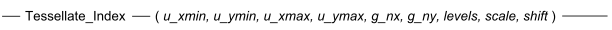# 16.20 - Syntax - Teradata Vantage NewSQL Engine

## Teradata Vantage™ Geospatial Data Types

prodname
vrm_release
16.20
created_date
March 2019
category
Programming Reference
featnum
B035-1181-162Ku_xmin
The coordinates of the universe of interest.

The data type is FLOAT.

u_ymin
The coordinates of the universe of interest.

The data type is FLOAT.

u_xmax,
The coordinates of the universe of interest.

The data type is FLOAT.

u_ymax
The coordinates of the universe of interest.

The data type is FLOAT.

g_nx
The number of grid cells to divide the universe into in the X dimensions.

The data type is INTEGER.

g_ny
The number of grid cells to divide the universe into in the Y dimensions.

The data type is INTEGER.

levels
The number of levels in the two-dimensional grid. There are always levels + 1 levels, where level 0 is the entire universe. Higher levels are more granular.

The data type is INTEGER and the range of values is 1 to 15.

scale
The scaling factor between grid levels. For example, if g_nx * g_ny is 100x100 and level is 2 and scale is 0.1, you have a 3 level grid (100x100, 10x10, 1x1).

The data type of scale is FLOAT and the value must be greater than 0.0 and less than 1.0.

shift
An INTEGER value that represents the number of times to shift the grid at each level. If the value of shift is 0, the method performs no shifting. If the value of shift is 1, the method creates four grids for each level, where it shifts each of the four grids in a unique manner.

Shifting the grid helps to avoid assigning smaller spatial objects to large cells if they cross a grid cell boundary.